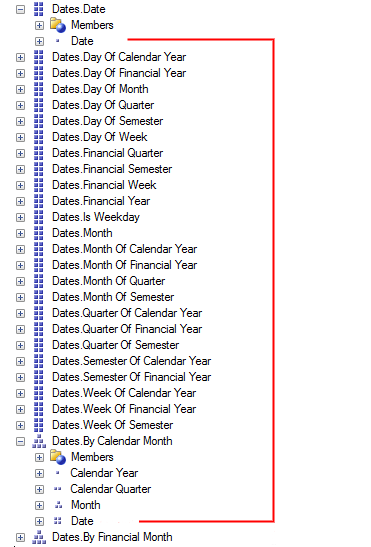# MDX: Month to date with LinkMember

The LinkMember function of MDX can be extremely useful especially when developing parameter driven reports.

I recently had to create a report that took the current date as a parameter and was then required to display month to date values. The date dimension had two hierarchies for Calendar and Financial months, which means I should be able to easily get the first day of the month using .Parent.FirstChild from my date parameter.

Unfortunately my date was in the format of a date dimension member: [Dates].[Date].& which is not a leaf member of the Calendar Month hierarchy. This is where LinkMember becomes useful. It takes my date in the format: [Dates].[Date].& and converts it to the corresponding leaf level in the Calendar hierarchy (or any other relevant hierarchy) [Dates].[By Calendar Month].[Date].&. The image below explains visually exactly what members are getting linked:So to get the first day of the month I can use the following query:

``````SELECT
[Measures].[SomeMeasure] ON COLUMNS,
[Dates].[Date].[Date].Members ON ROWS
FROM ( SELECT (
LinkMember([Dates].[Date].&, [Dates].[By Calendar Month]).Parent.FirstChild ) ON COLUMNS
FROM [SomeCube])
``````

This gives me the measure for the first day of the month no matter what the original date was. Once the LinkMember function is working the way we want it’s then a simple step to get all the days of the month to the date parameter by simply querying the set of {StartDate : EndDate}

`````` SELECT
[Measures].[SomeMeasure] ON COLUMNS,
[Dates].[Date].[Date].Members ON ROWS
FROM ( SELECT ( {